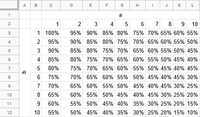# Equation Needed to Find Probability Based on 2 10-Digit Numbers

#### jvderosa94

##### New member
I'm trying to find an equation to calculate a probability based on 2 ten digit numbers. I'm terrible at math and don't really know how to word it, so I created an excel spreadsheet showing the relationship between the two numbers. It's very linear and should be very easy to do (I would imagine).The equation should take two numbers, a and b (letters aren't important) and should output the percentage.

#### ksdhart2

##### Senior Member
Reading down the first column shows that the percentage decreases by 5 points for each one unit we increment $$b$$ by. Likewise, reading across the first row shows that the percentage decreases by 5 points for each unit we increment $$a$$ by. Based on this, what can you say about how much the percentage would decrease if you incremented both $$a$$ and $$b$$ by one unit each? What would happen to the percentage if you incremented $$a$$ by 5 units and $$b$$ by 3 units? What would happen if you increased $$a$$ by 7 units and $$b$$ by 8 units? Where does all of this lead you?

#### Harry_the_cat

##### Senior Member
You say: "I'm trying to find an equation to calculate a probability based on 2 ten digit numbers."

Do you mean 2 digit numbers?

Also should the row and column headed "10" really be "0"?